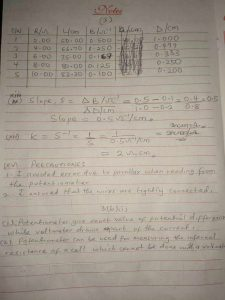NECO Physics Practical 2020 Questions and Answers

(1)
Graph1bi)
(i)The have the same magnitude/size
(ii)They can be obtained/calculated by the same means

(1bii)(1bii)
T²= 9²+12²
T= √81+144
T= √225
T= 15N

(3)
Tabulate
S/N; 1|2|3|4|5|

R/Ω; 2.00|4.00|6.00|8.00|10.00|

L/cm; 50.00|66.70|75.00|80.00|83.30|

B/Ω^-¹; 0.500|0.250|0.167|0.125|0.100|

Δ/cm; 1.000|0.499|0.333|0.250|0.200|

(3xiii)
Slope ,S=ΔB/Ω^-¹/ΔΔ/cm =0.5-0.1/1.0-0.2=0.4/0.8=0.5
Slope =0.5Ω^-¹/cm

(3xiv)
K=S^-¹= 1/5=1/0.5Ω^-¹/cm =2Ωcm

(3xv)
-precautions-
(i) I avoided error due to parallax when reading from the potentiometer
(ii) I ensured that the wire are tightly connected.

(3)
Graph(3bi)
(i) potentiometer give exact value of potential difference while voltmeter draws apart of the current
(ii) potentiometer can be used for measuring the internal resistance of a cell which cannot be done with voltmeter

(3bii)
x/R= c/100-c
x/R= 20-0/100-20
x/20= 20/80
x= 20*20/80
x= 400/80
x= 5Ω

## NECO Physics Practical 2020 Questions and Answers

This site uses Akismet to reduce spam. Learn how your comment data is processed.